2D Heat transfer - part 2

Published: 1st June, 2018

This second part looking at the difference between heat loss calculated in 1D and that calculated by Agros2D in 2D explores slightly more complex geometry. I hope to gain some insight into a couple of key questions:

1. The thermal model in MyHomeEnergyPlanner is based on internal element dimensions and does therefore not take into account heat lost through corners. I'd like to get an idea for the likely degree of error introduced by taking this simplification.

2. Where its not possible to fully insulate all external surfaces of a wall, what is the effect on overall heat loss of sections left uninsulated?

3. Similar to the last but in relation to the mid terrace house project. In a terrace house if the external wall insulation does not continue over the house boundary with the neighbouring house how much heat do we loose through this uninsulated pathway? (Assuming the neighbouring house is heated to the same internal temperature).

Model 1: Four-sided building 2D vs 1D

Modelling a four sided 2D building as below using a triangular - quad join mesh and the number of refinements set to 4 and polynomial order set to 5. I get the following results:

• 2D Heat loss from Agros2D: 394.9 Watts
• 1D Heat loss 1.7 W/K.m2 x 5m2 x 11K x 4 sides: 374.0 Watts
• 2D heat loss result is 5.6% larger than the 1D calculation.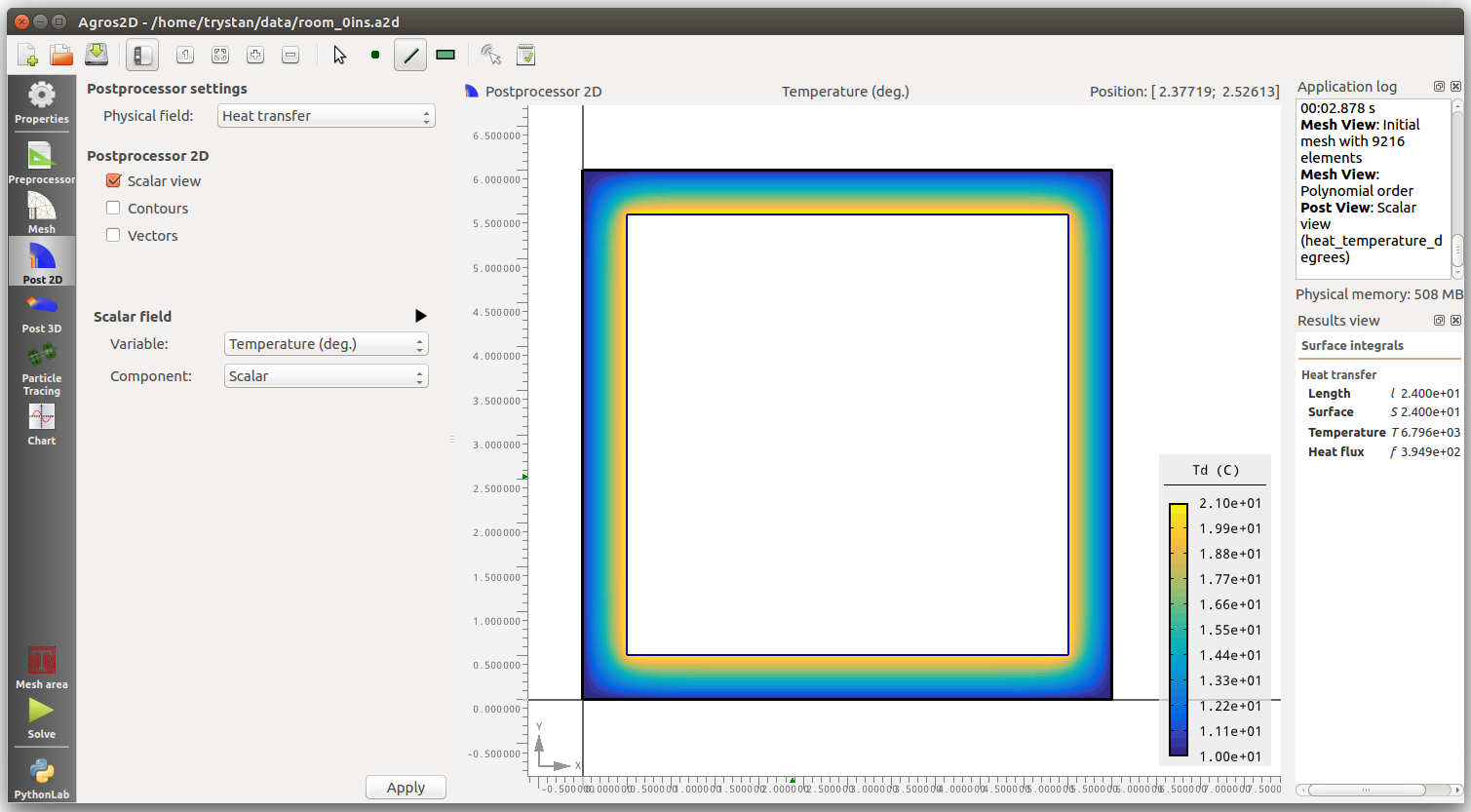Model 2-5: 100mm Wood Fibre External Insulation

These next four model runs explore the effect of partially insulating an external wall externally. Perhaps there is a requirement to keep the appearance of the front of a building or that detailing on one side is too complex, what is the value of insulating the walls that are easier to insulate? Does the existence of uninsulated adjacent walls reduce the benefit of the insulated wall significantly?

 One side insulated to 100mm 2D Heat loss from Agros2D: 320.7 Watts 1D Heat loss 1.7 W/K.m2 x 5m2 x 11K x 3 sides: 280.5 Watts 1D Heat loss 0.317 W/K.m2 x 5m2 x 11K x 1 side: 17.4 Watts Total 1D heat loss 280.5 + 17.4 = 297.9 Watts 2D heat loss result is 7.7% larger than the 1D calculation. 1D Heat loss reduction: 76.1W, 2D Heat loss reduction: 74.2W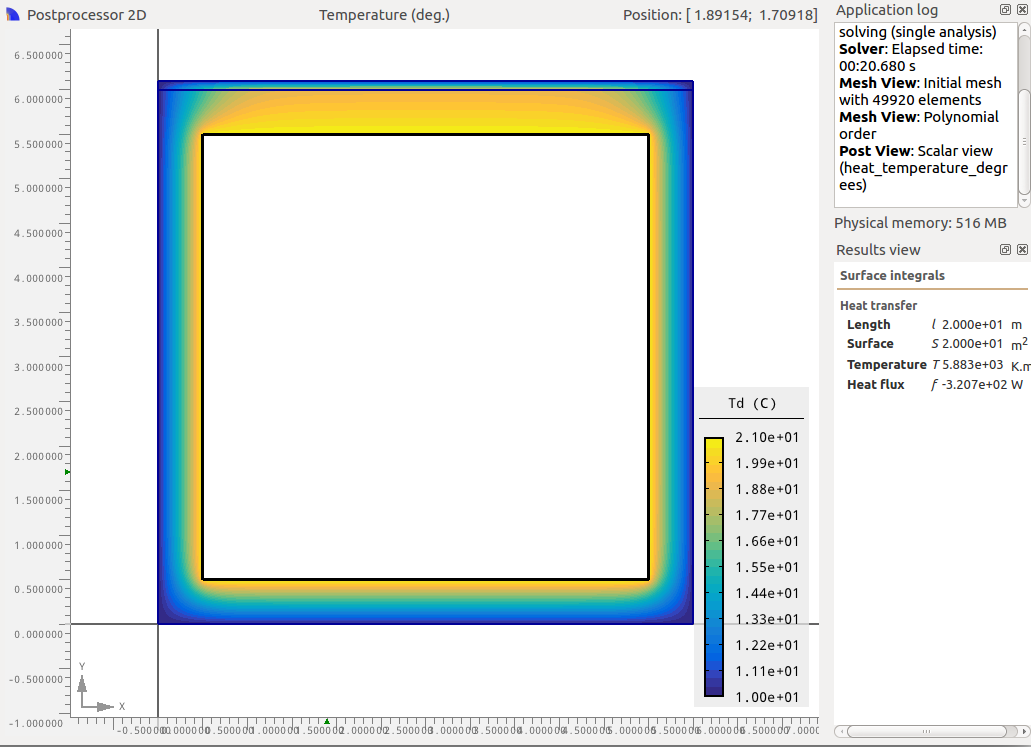Two sides insulated to 100mm 2D Heat loss from Agros2D: 242.3 Watts 1D Heat loss 1.7 W/K.m2 x 5m2 x 11K x 2 sides: 187 Watts 1D Heat loss 0.317 W/K.m2 x 5m2 x 11K x 2 side: 34.8 Watts Total 1D heat loss 93.5 + 52.2 = 221.8 Watts 2D heat loss result is 9.2% larger than the 1D calculation. 1D Heat loss reduction: 152.2W, 2D Heat loss reduction: 152.6W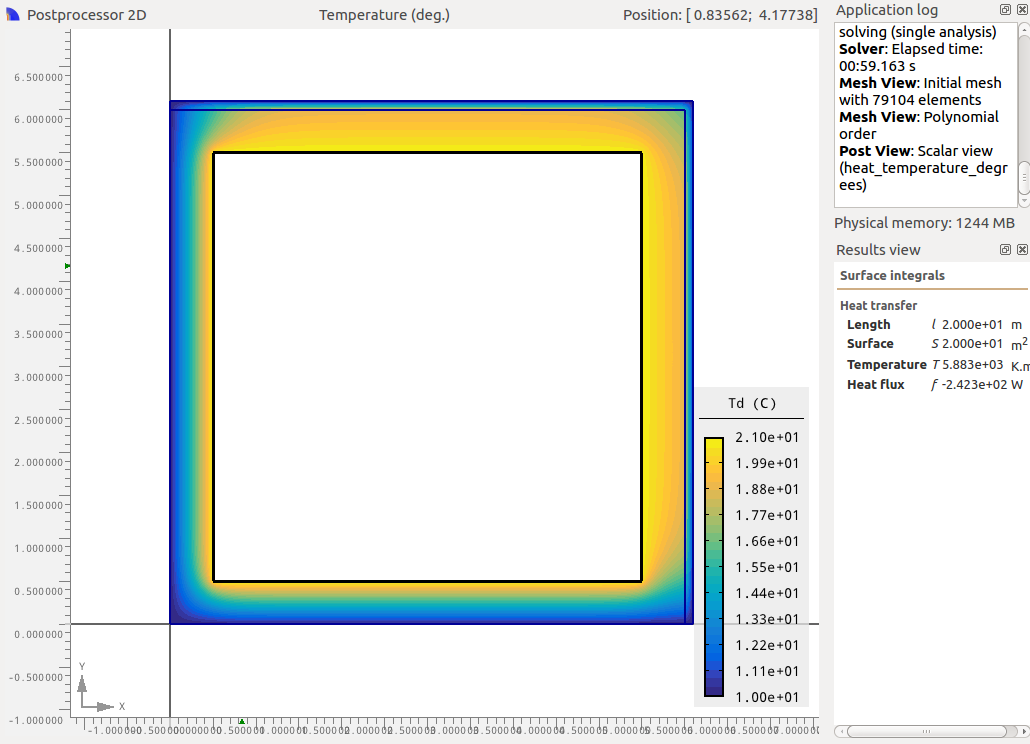Three sides insulated to 100mm 2D Heat loss from Agros2D: 163.9 Watts 1D Heat loss 1.7 W/K.m2 x 5m2 x 11K x 1 sides: 93.5 Watts 1D Heat loss 0.317 W/K.m2 x 5m2 x 11K x 3 side: 52.2 Watts Total 1D heat loss 93.5 + 52.2 = 145.7 Watts 2D heat loss result is 12.5% larger than the 1D calculation. 1D Heat loss reduction: 228.3W, 2D Heat loss reduction: 231W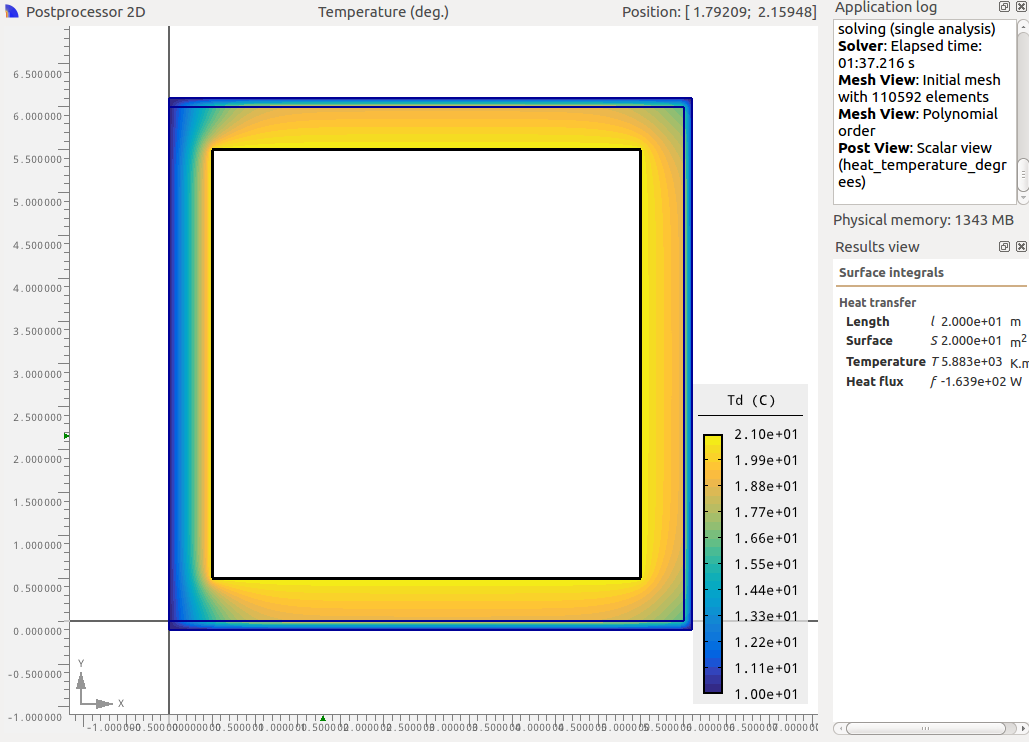Four sides insulated to 100mm 2D Heat loss from Agros2D: 80.7 Watts 1D Heat loss 0.317 W/K.m2 x 5m2 x 11K x 4 sides: 69.6 Watts 2D heat loss result is 15.9% larger than the 1D calculation. 1D Heat loss reduction: 304.4W, 2D Heat loss reduction: 314.2W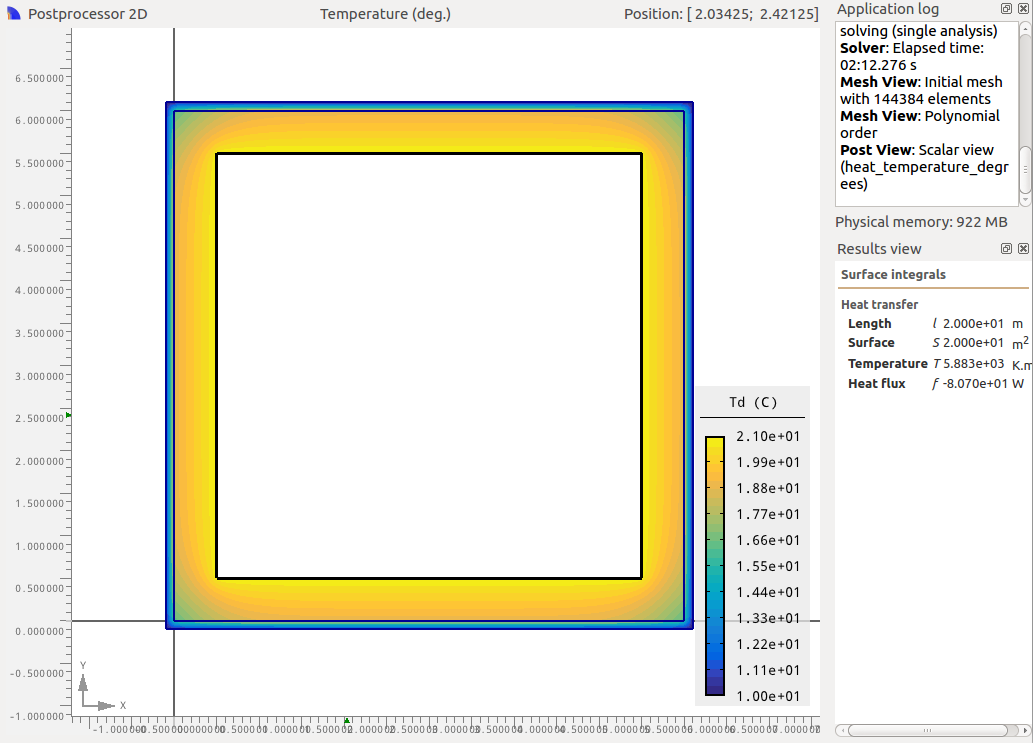The 2D model suggests that much of the value of partially insulating the walls that are possible to insulate is maintained. While the 2D heat loss is larger in all cases than the 1D heat loss by between 7.7% and 15.9% the heat loss reduction from the base case of four uninsulated walls are very similar in between all respective 1D:2D cases with a difference of between -2.5% and 3.2%.

Model 6-9: 100mm Wood Fibre Internal Insulation

Is there a significant difference if the walls are internally insulated instead?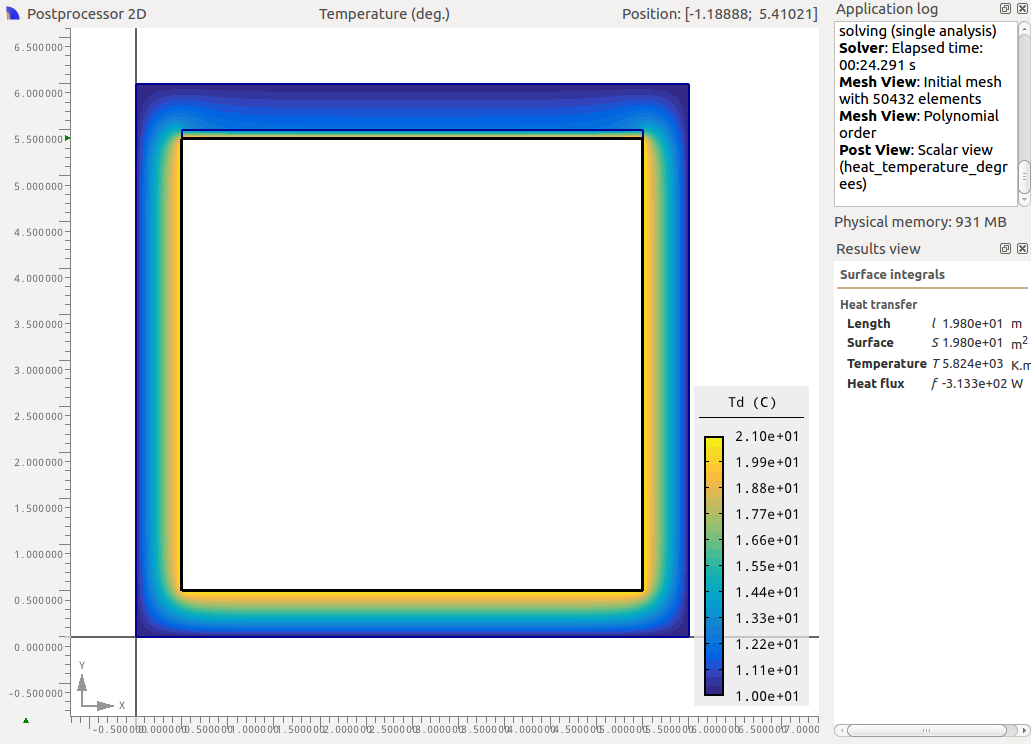One side insulated to 100mm 2D Heat loss from Agros2D: 313.3 Watts 1D Heat loss 1.7 W/K.m2 x 5m2 x 11K x 3 sides: 280.5 Watts 1D Heat loss 0.317 W/K.m2 x 5m2 x 11K x 1 side: 17.4 Watts Total 1D heat loss 280.5 + 17.4 = 297.9 Watts 2D heat loss result is 5.2% larger than the 1D calculation. 1D Heat loss reduction: 76.1W, 2D Heat loss reduction: 81.6W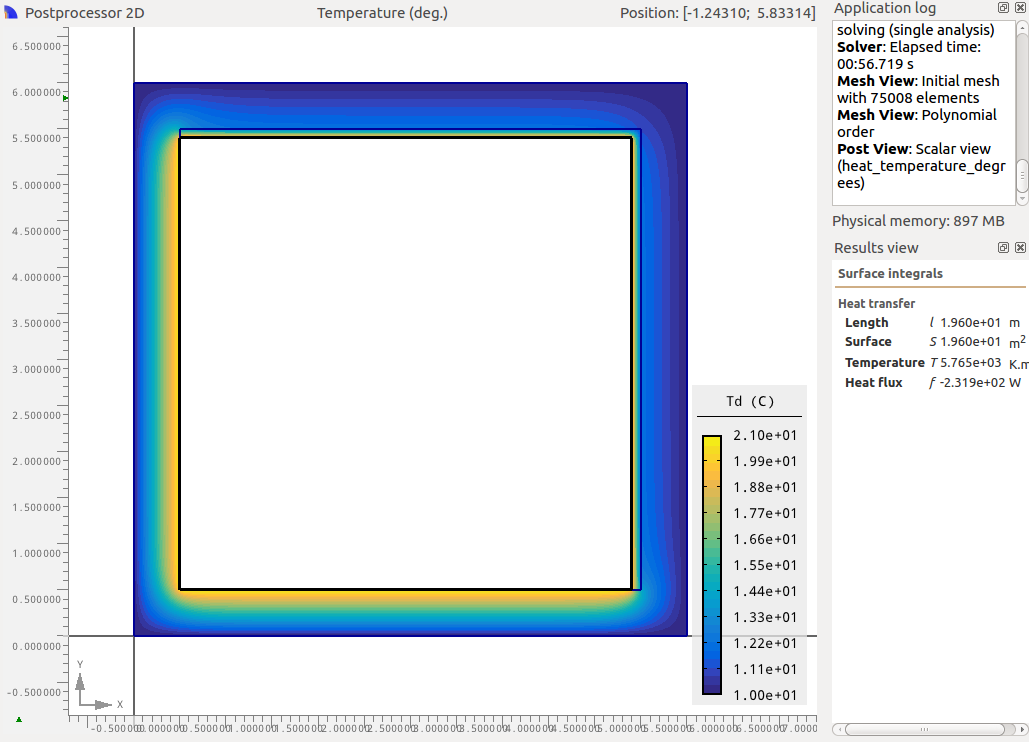Two sides insulated to 100mm 2D Heat loss from Agros2D: 231.9 Watts 1D Heat loss 1.7 W/K.m2 x 5m2 x 11K x 2 sides: 187 Watts 1D Heat loss 0.317 W/K.m2 x 5m2 x 11K x 2 side: 34.8 Watts Total 1D heat loss 93.5 + 52.2 = 221.8 Watts 2D heat loss result is 4.6% larger than the 1D calculation. 1D Heat loss reduction: 152.2W, 2D Heat loss reduction: 163.0W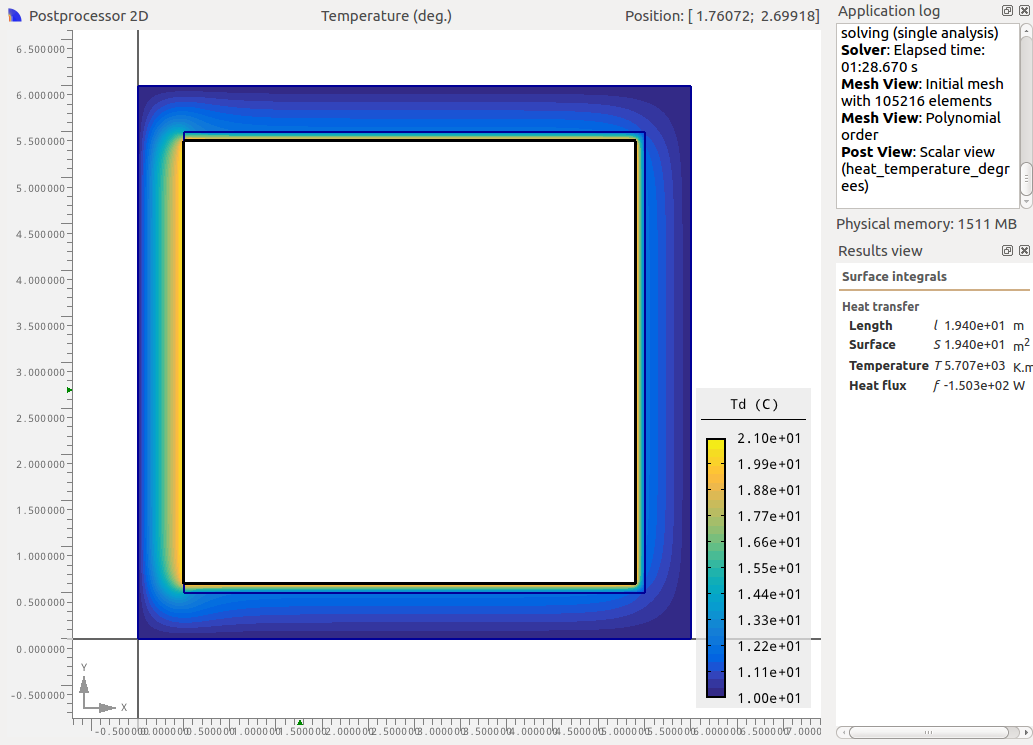Three sides insulated to 100mm 2D Heat loss from Agros2D: 150.3 Watts 1D Heat loss 1.7 W/K.m2 x 5m2 x 11K x 1 sides: 93.5 Watts 1D Heat loss 0.317 W/K.m2 x 5m2 x 11K x 3 side: 52.2 Watts Total 1D heat loss 93.5 + 52.2 = 145.7 Watts 2D heat loss result is 3.2% larger than the 1D calculation. 1D Heat loss reduction: 228.3W, 2D Heat loss reduction: 244.6W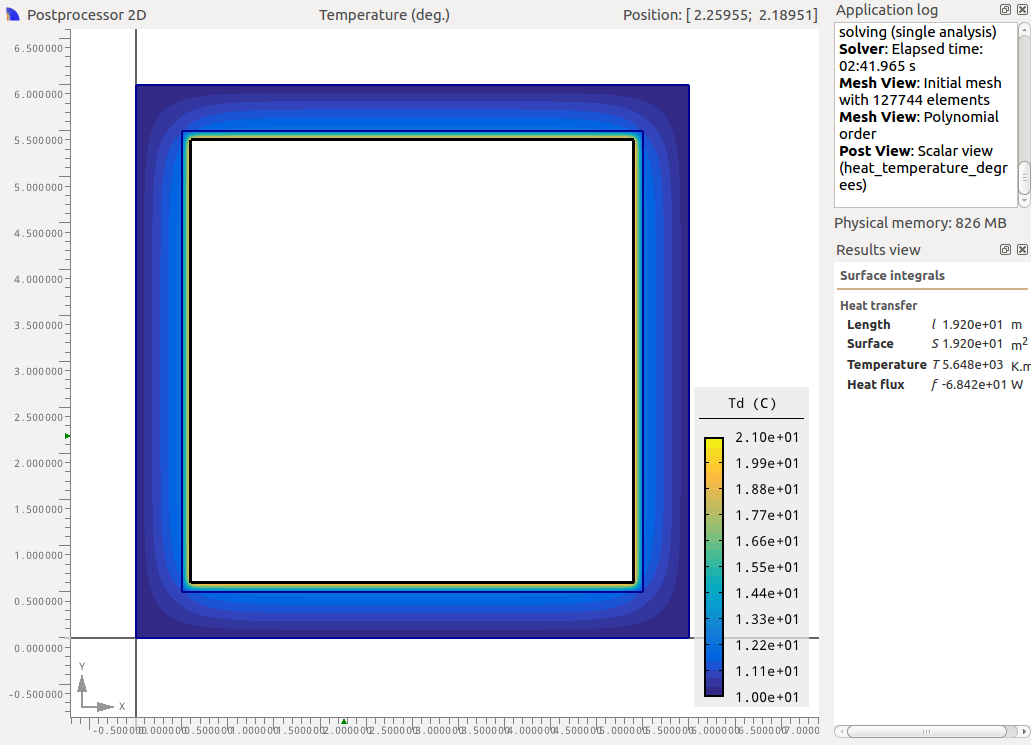Four sides insulated to 100mm 2D Heat loss from Agros2D: 68.4 Watts 1D Heat loss 0.317 W/K.m2 x 5m2 x 11K x 4 sides: 69.6 Watts 2D heat loss result is 1.7% less than the 1D calculation. 1D Heat loss reduction: 304.4W, 2D Heat loss reduction: 326.5W

The contrast with the externally insulated case is interesting, rather than a trend towards a larger difference between the 1D result and the 2D result we see the difference decrease as we insulate more of the internal walls. The heat loss is also lower than the externally insulated examples. The externally insulated wall looses 18% more heat than the internally insulated wall when all four walls are insulated.

The inside perimeter of the insulation layer in the internal insulation case is 19.2m while it is 24m in the external insulation case. There is more area for the heat to be lost through in the external insulation case.

Model 10-14 Terrace House

In a terrace house if the external wall insulation does not continue over the house boundary with the neighbouring house how much heat do we loose through this uninsulated pathway? (Assuming the neighbouring house is heated to the same internal temperature).

 Uninsulated 2D Heat loss from Agros2D: 246.4 Watts 1D Heat loss (1.6+3.3+2.4+4.5)×1.7×11 = 220.7 Watts 2D heat loss result is 12% larger than the 1D calculation.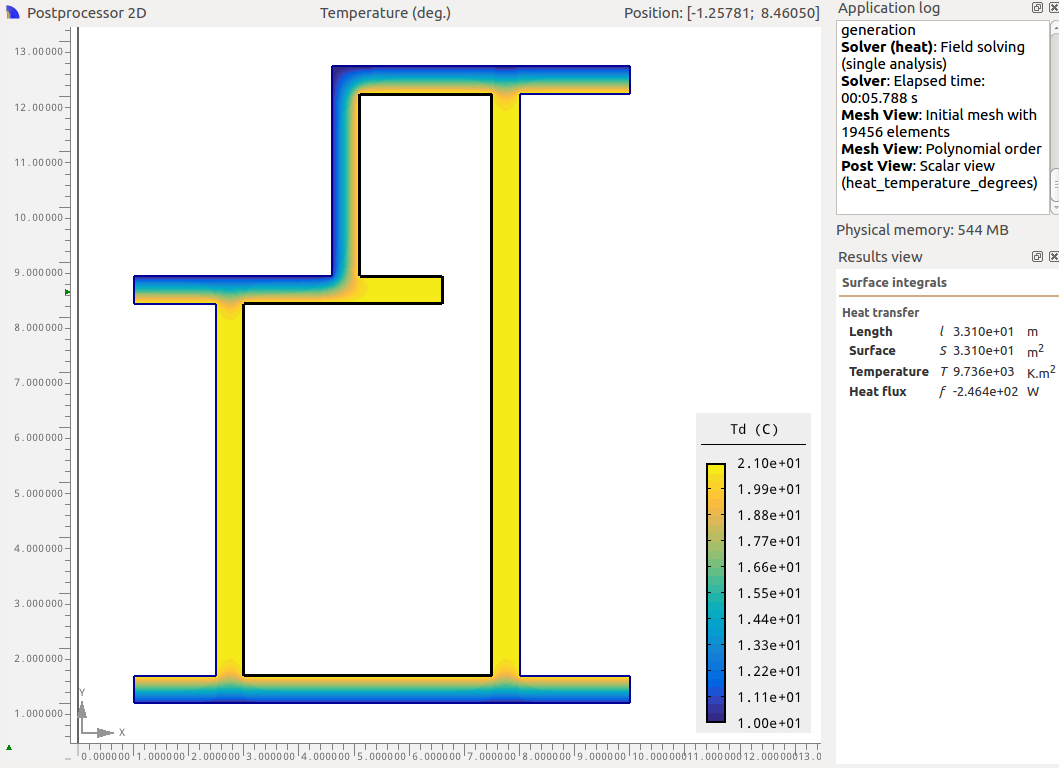External Insulation up to house boundary 2D Heat loss from Agros2D: 62.37 Watts 1D Heat loss (1.6+3.3+2.4+4.5)×0.317×11 = 41.1 Watts 2D heat loss result is 52% larger than the 1D calculation.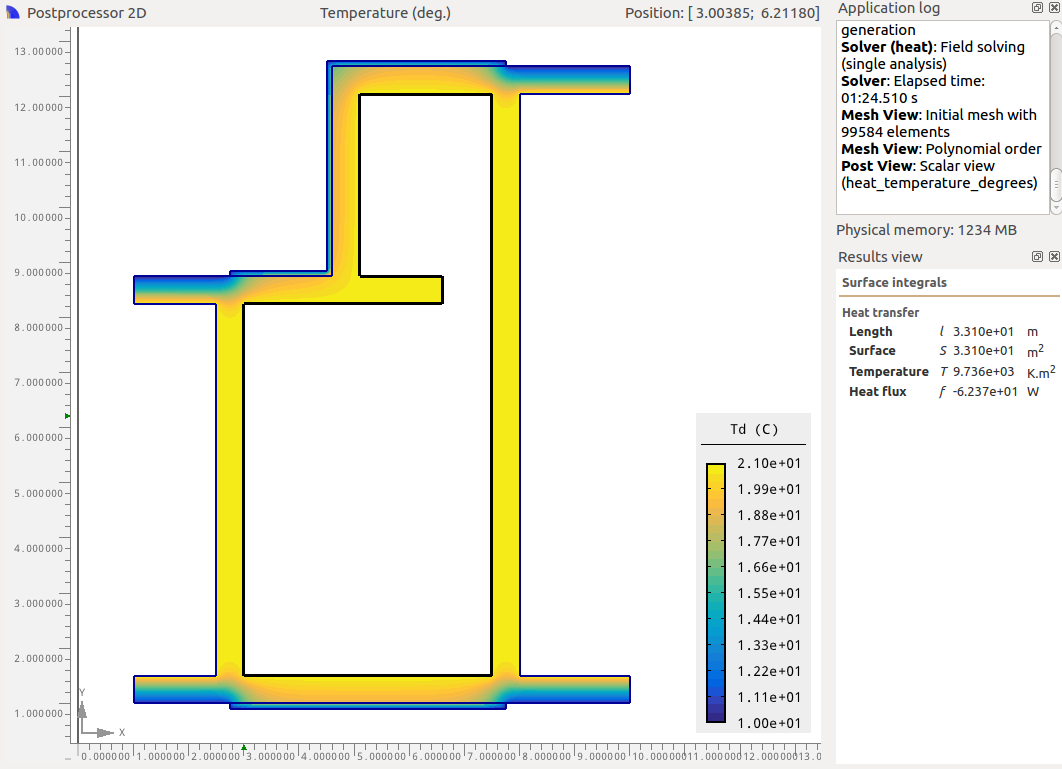External Insulation overlapping house boundary 2D Heat loss from Agros2D: 54.92 Watts 1D Heat loss (1.6+3.3+2.4+4.5)×0.317×11 = 41.1 Watts 2D heat loss result is 34% larger than the 1D calculation.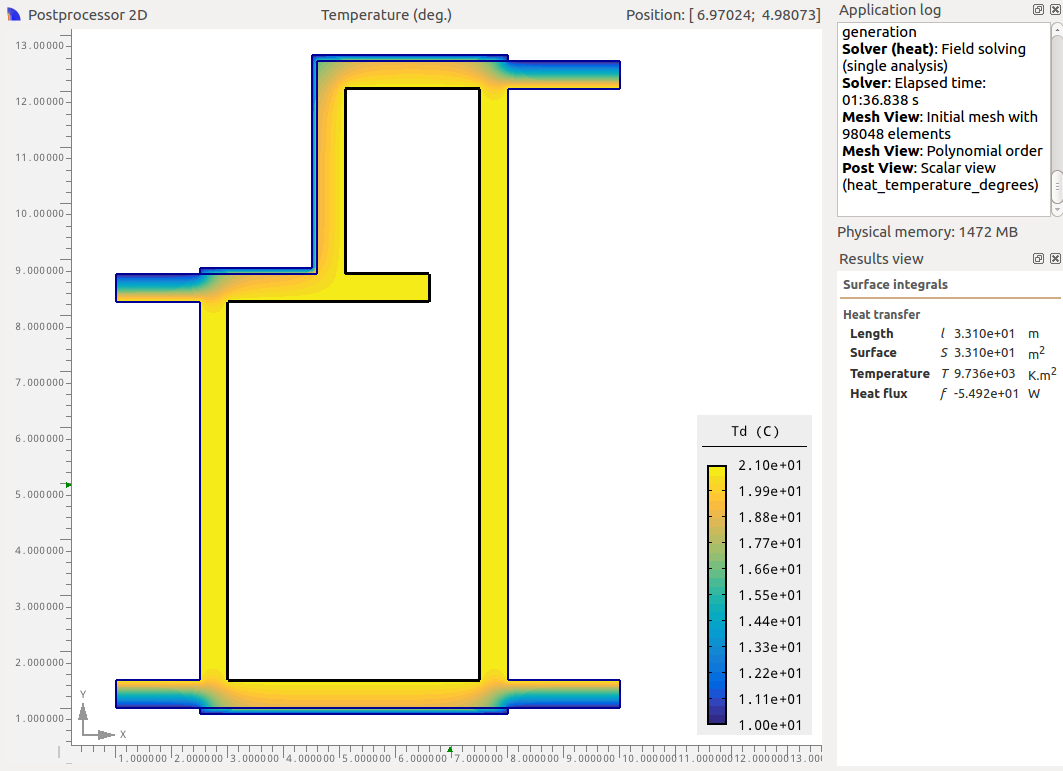Full Terrace External Insulation 2D Heat loss from Agros2D: 46.93 Watts 1D Heat loss (1.6+3.3+2.4+4.5)×0.317×11 = 41.1 Watts 2D heat loss result is 14% larger than the 1D calculation.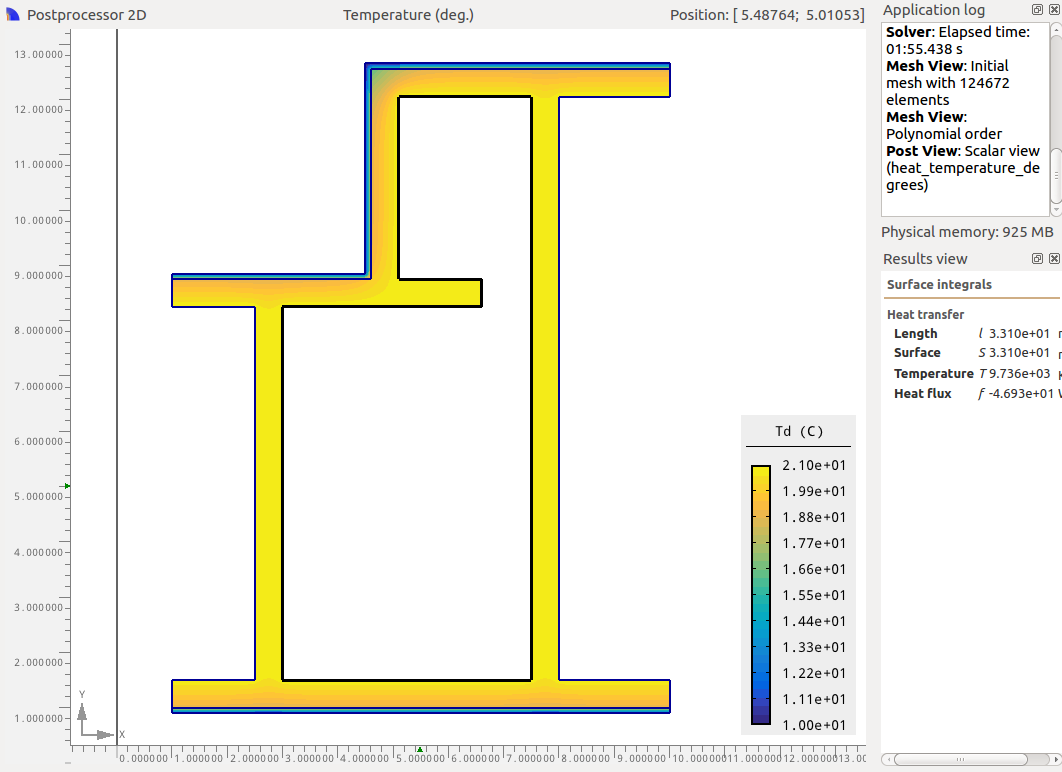Internal Insulation 2D Heat loss from Agros2D: 60.53 Watts 1D Heat loss (1.6+3.3+2.4+4.5)×0.317×11 = 41.1 Watts 2D heat loss result is 47% larger than the 1D calculation.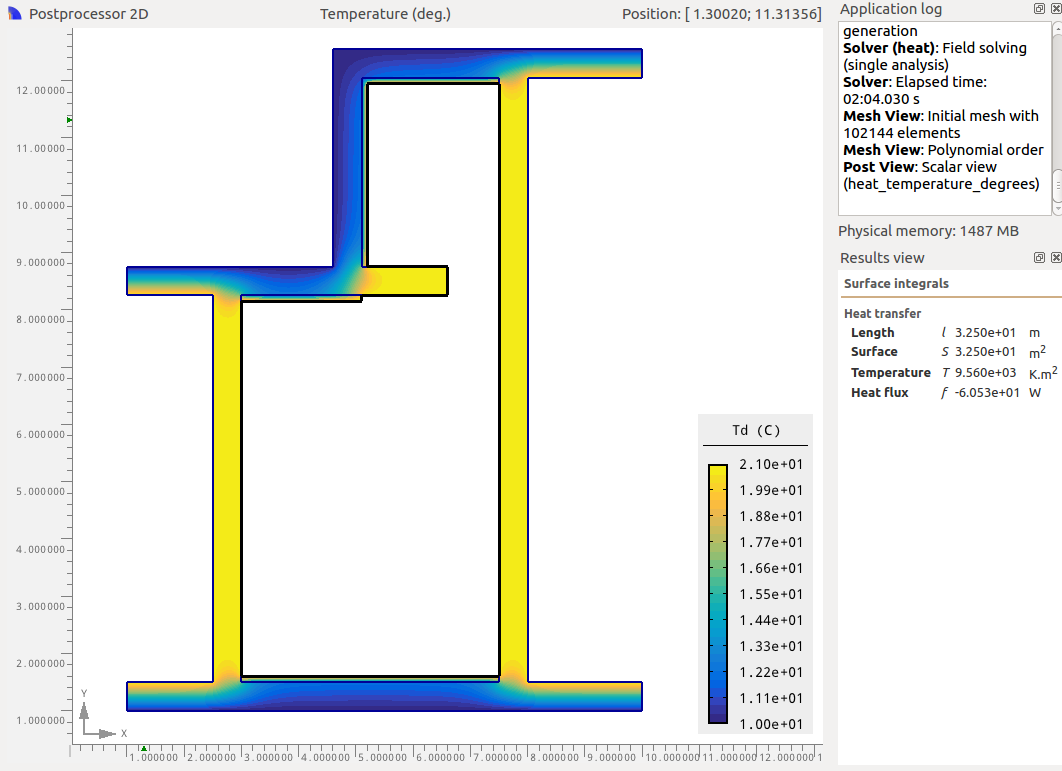Internal Insulation extended 2D Heat loss from Agros2D: 52.17 Watts 1D Heat loss (1.6+3.3+2.4+4.5)×0.317×11 = 41.1 Watts 2D heat loss result is 27% larger than the 1D calculation.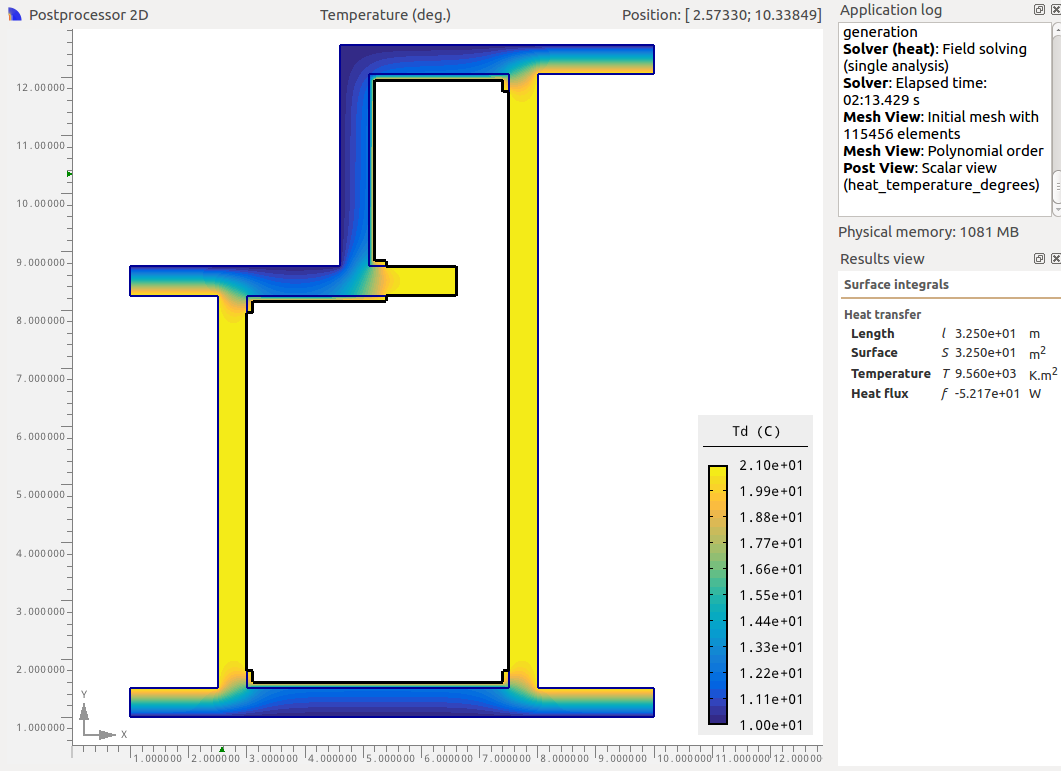These results suggest significant under-calculation of heat loss in the 1D case due to its inability to take into account both the complexity of the geometry and the effect of thermal bridging. Apart from the full terrace external insulation option. Partial internal insulation outperforms the partial external insulation in both cases.

The key take away for me is that the benefit of insulating is still just as significant even after taking into account the effects of the complex geometry and thermal bridging. Partly because while the insulated heat loss is higher in the 2D case the starting point is also higher and so the overall reduction in heat loss is similar or actually better 1D: 180W heat loss reduction vs 2D: 184-200W

There are many more factors to consider as ever when choosing between internal and external insulation including key issues such as interstitial condensation, due to the presence of a due point inside internal insulated walls that can rot joists that penetrate through the internal insulation.